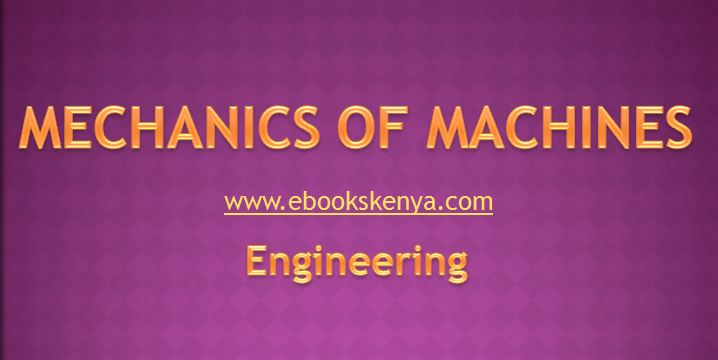# Mechanics of Machines KNEC notesIntroduction

Mechanics of machines deals with forces, motion and power of machines in motion like hoists and vehicles.

The recommended instructional approach is that which will emphasize on experiments, industrial visits and analysis of various mechanical principles.

### Kinematics

• Definition of kinematics of a particle
• Equations of motion
• Application of equations of motion
• Derivation from first principles expression for centripetal acceleration of a particle moving with uniform angular velocity

### Impulse and Momentum

• Definition of linear momentum
• Explanation of the relationship between force and momentum of a body
• Explanation of linear  impulse
• Explanation of the relationship between linear impulse and linear momentum of a body
• Solution of problems in linear momentum and linear impulse
• Explanation of angular momentum
• Derivation from first principles, equations of angular momentum and impulse
• Solution of problems on angular momentum and impulse
• Verification of the conservation of momentum

### Mass Moments of Inertia

• Explanation of axial moment of inertia  of a mass
• Statement of expression for mass moment of inertia of an element about three mutually perpendicular axes
• Derivation of expressions of centroidal mass moment of inertia (common regular objects)
• Explanation of polar moment of inertia
• Application of expressions to solve problems
• Centroidal mass moment of inertia for  common regular shapes
• Statement of parallel axes theorem
• Explanation of parallel axes theorem
• Application of parallel axes theorem
• Definition of radius of gyration
• Application of expression of radius of gyration to solve problems

### Area Of Moment of Inertia

• Explanation of axial moment of inertia  of an area
• Elemental area rotated about an axis perpendicular to its plane
• Derivation of an expression for polar moment of inertia of an area
• Explanation of the product of inertia
• Derivation of an expression for the product of inertia of an area
• Statement of parallel axis theorem
• Diagram
• Application of inertia expressions to solve problems in:
• regular areas
• composite areas
• Explanation of moments of inertia of any (x, y) with respect to rotated set of axis
• Statement of expressions for moment of inertia of an area with rotated axes
• Application of Mohr’s cycle to solve  problems

### Belts and Clutches

• Identification of common types of belts
• Derivation of belt equations
• Application of equation to solve belt problems
• Identification of common clutches
• Derivation of clutch equations
• Application of the equations to solve clutch problems
• Ratio of belt tension
• Coefficient of friction between belt and pulley
• Torque in clutches
• Coefficient of friction in clutches

### Geared Systems

• Description of different types of gear drives
• Derivation of equations for gear drives
• Application of the equations to solve gear drive problems
• Torque in geared systems
• Efficiency in geared systems
• Dynamics of a Rigid Body in Translation
• Definition of dynamics of a rigid body
• Statement of the scalar equation for translation
• Moment of external forces about mass centre of a body
• Application of the equation to solve problems

### Dynamics of Rigid Body in Rotation

• Identification of rotation of a body about a non-centroidal axis
• statement of equation of motion for  rotation about a non-centroidal axis
• Balancing of masses

### Dynamics of Rigid Body in Plane

• Statement of plane motion
• Explanation of equations of plane motion
• Application of equation of plane motion
(Visited 2,961 times, 1 visits today)

## 2 thoughts on “Mechanics of Machines KNEC notes”

1.luke says:

How can i access these and other notes, its urgent please

2.Katana .menza says:

This notes are useful for me